# Ex.6.3 Q1 Triangles Solution - NCERT Maths Class 10

Go back to  'Ex.6.3'

## Question

State which pairs of triangles in Figure are similar. Write the similarity criterion used by you for answering the question and also write the pairs of similar triangles in the symbolic form:

1)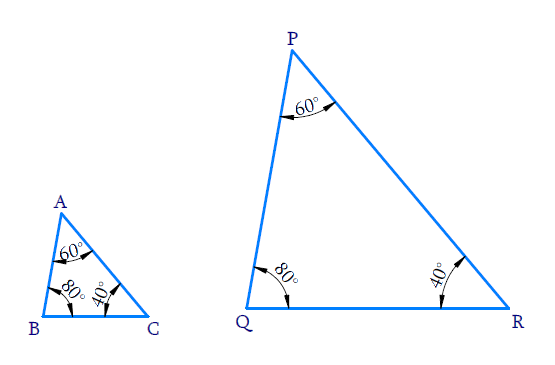## Text Solution

Reasoning:

As we know if in two triangles, corresponding angles are equal, then their corresponding sides are in the same ratio (or proportion) and hence the two triangles are similar.

This is referred as $$AAA$$ (Angle–Angle–Angle) criterion of similarity of two triangles.

Steps:

In $$\Delta ABC$$ and $$\Delta PQR$$

\begin{align} \angle A = \angle P = 60{}^ \circ \\ \angle B = \angle Q = {80^ \circ } \\ \angle C = \angle R = {40^ \circ } \\ \end{align}

All the corresponding angles of the triangles are equal.

By $$AAA$$ criterion \begin{align}\Delta ABC \sim \Delta PQR\end{align}

2)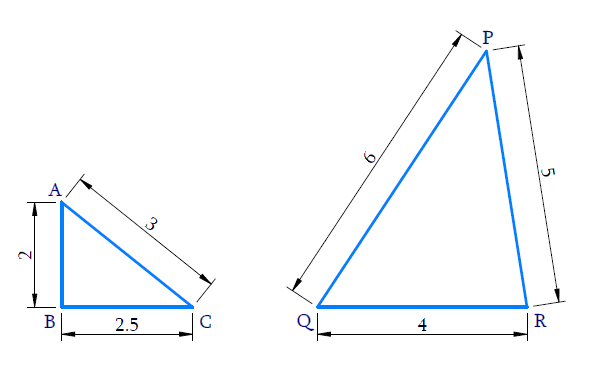Reasoning :

As we know if in two triangles, side of one triangle are proportional to (i.e., in the same ratio ) the sides of the other triangle, then their corresponding angles are equal and hence the two triangles are similar.

This is referred as $$SSS$$ (Side–Side–Side) similarity criterion for two triangles.

Steps:

$$\rm{ In} \Delta ABC\, {\rm{and}}\,\Delta QRP$$

\begin{align} \frac{{AB}}{{QR}} &= \frac{2}{4} = \frac{1}{2} \\ \frac{{BC}}{{PR}} &= \frac{{2.5}}{5} = \frac{1}{2} \\ \frac{{AC}}{{PQ}} &= \frac{3}{6} = \frac{1}{2} \ \\ \Rightarrow \frac{{AB}}{{QR}} &= \frac{{BC}}{{PR}} = \frac{{AC}}{{PQ}} = \frac{1}{2} \\ \end{align}

All the corresponding sides of two triangle are in same proportion.

By $$SSS$$ criterion $$\Delta ABC \sim \Delta QPR$$

3)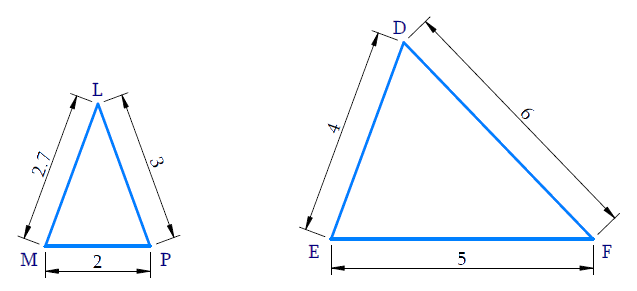Reasoning:

As we know if in two triangles, side of one triangle are proportional to (i.e., in the same ratio ) the sides of the other triangle, then their corresponding angles are equal and hence the two triangles are similar.

This is referred as $$SSS$$ (Side–Side–Side) similarity criterion for two triangles.

Steps:

In $$\Delta LMP$$ and  $$\Delta FED​​​​​​​$$

\begin{align}\frac{LM}{FE}& =\frac{2.7}{5} \\ \frac{MP}{ED}& =\frac{2}{4}=\frac{1}{2} \\ \frac{LP}{FD}&=\frac{3}{6}=\frac{1}{2} \\ \Rightarrow \frac{LM}{FE} &\ne \frac{MP}{ED}\,\,=\,\frac{LP}{FD} \end{align}

All the corresponding sides of the two triangles are not in the same proportion.

Hence triangles are not similar.

$$\Delta LMP \nsim \Delta FED$$

4)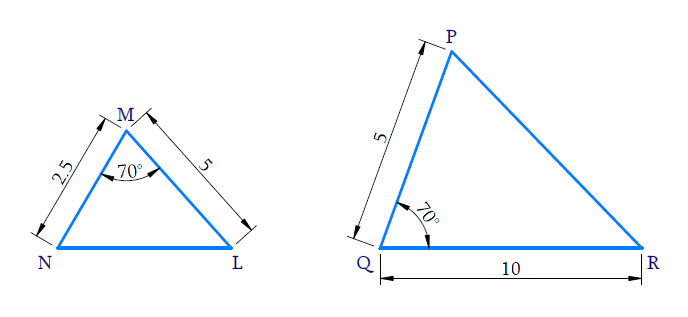Reasoning:

As we know if one angle of a triangle is equal to one angle of the other triangle and the sides including these angles are proportional, then the two triangles are similar.

This criterion is referred to as the $$SAS$$ (Side–Angle–Side) similarity criterion for two triangles.

Steps:

In $$\Delta NML$$ and $$\Delta PQR$$

\begin{align} & \,\,\,\,\,\,\,\frac{NM}{PQ}=\frac{2.5}{5}=\frac{1}{2} \\ & \,\,\,\,\,\,\,\,\frac{ML}{QR}=\frac{5}{10}=\frac{1}{2} \\ & \Rightarrow \frac{NM}{PQ}=\frac{ML}{QR}=\frac{1}{2} \\ & \,\,\,\,\,\,\,\angle M=\angle Q={{70}^{0}} \\ \end{align}

One angle of a triangle is equal to one angle of the other triangle and the sides including these angles are proportional.

By $$SAS$$ criterion $$\Rightarrow \,\,\,\Delta NML\sim \Delta PQR$$

5)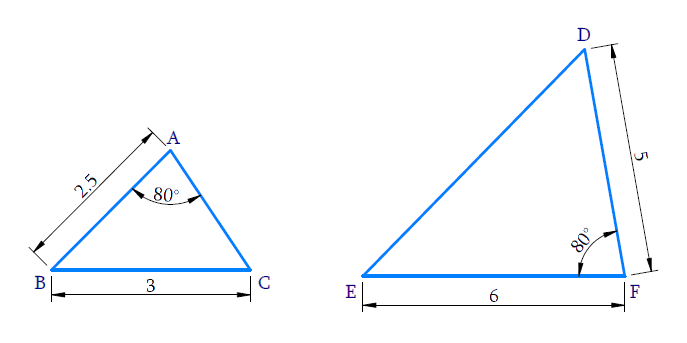Reasoning:

As we know if one angle of a triangle is equal to one angle of the other triangle and the sides including these angles are proportional, then the two triangles are similar.

This criterion is referred to as the $$SAS$$ (Side–Angle–Side) similarity criterion for two triangles.

Steps:

In $$\Delta A B C,$$and $$\Delta D F E$$

\begin{align} & \frac{AB}{DF}=\frac{2.5}{5}=\frac{1}{2} \\ & \frac{BC}{EF}=\frac{3}{6}=\frac{1}{2} \\ & \frac{AB}{DF}=\frac{BC}{EF}=\frac{1}{2} \end{align}

$\angle A=\angle F={{80}^{\circ }}$

But $$\angle B$$ must be equal to $${{80}^{0}}$$

$$\because$$ The sides $$AB, \,BC$$ includes $$\angle B$$, not $$\angle A$$

Therefore, $$SAS$$ criterion is not satisfied

Hence,the triangles are not similar,$$\Delta ABC \nsim \Delta DFE$$

6)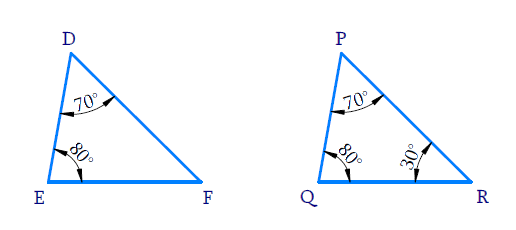Reasoning:

As we know if one angle of a triangle is equal to one angle of the other triangle and the sides including these angles are proportional, then the two triangles are similar.

This criterion is referred to as the $$AAA$$ (Angle–Angle–Angle) criterion of similarity of two triangles

Steps:

In $$\Delta DEF$$

$\angle D={{70}^{0}};\angle E={{80}^{0}}$

$$\Rightarrow \angle F={{30}^{0}}$$ $$[ \because$$ Sum of the angles in a triangle is $${{180}^{0}}$$] )

Similarly,

In $$\Delta PQR$$

$\angle Q={{80}^{\circ }};\,\,\,\,\,\angle R={{30}^{\circ }}\,\,\\ \Rightarrow \angle P={{70}^{\circ }}$

In $$\Delta \,DEF\,\rm{and}\,\Delta \,PQR$$

\begin{align} & \angle D=\angle P=70{}^{0} \\ & \angle E=\angle Q={{80}^{0}} \\ & \angle F=\angle R={{30}^{0}} \\ \end{align}

All the corresponding angles of the triangles are equal.

By $$AAA$$ criterion

$$\Rightarrow \,\,\Delta DEF\sim \Delta PQR$$

Alternate method:

Reasoning:

As we are ware if two angles of one triangle are respectively equal to two angles of another triangle, then the two triangles are similar.

This may be referred to as the $$AA$$  criterion for two triangles.

Steps:

In $$\Delta DEF$$

$\angle D={{70}^{0}};\,\,\angle E={{80}^{0}}$

$$\Rightarrow \angle F={{30}^{0}}$$  [$$\because$$ Sum of the angles in a triangle is $${{180}^{0}}]$$

Now ,In $$\,\Delta DEF \,\rm{and}\, \Delta PQR$$

\begin{align}{\angle E}&={\angle Q=80^{\circ}} \\ {\angle F}&={\angle R=30^{\circ}} \end{align}

Pair of corresponding angles of the triangles are equal.

By $$AA$$ criterion $$\,\Delta DEF \,\sim\, \Delta PQR$$

Learn from the best math teachers and top your exams

• Live one on one classroom and doubt clearing
• Practice worksheets in and after class for conceptual clarity
• Personalized curriculum to keep up with school